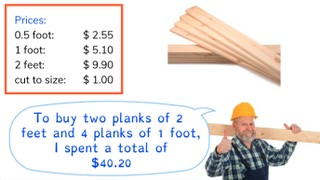Solving math problems with multiple operations

# Solving math problems with multiple operations8,000 schools use Gynzy92,000 teachers use Gynzy1,600,000 students use Gynzy

## General

Students learn to calculate with multiple operations.

## Standards

CCSS.Math.Content.5.OA.A.1
CCSS.Math.Content.5.OA.A.2

## Learning objective

Students will be able to solve problems with multiple operations.

## Introduction

Ask students to solve a set of division problems within the given time. Then start the timer again and ask students to solve the set of multiplication problems. You can also adjust the amount of time in the hourglass to better fit your classroom needs.

## Instruction

Explain to students that there are rules about the order of operations within a math problem. One of the rules is that x and ÷ are done before + and - . That means that all multiplication and division actions happen before the addition or subtraction actions. Show the problem 6 × 30 + 4 × 100. Show that to solve this problem you first solve the multiplication portion, and then add the numbers together. Show the next problem and read the story. Remind students to read the story carefully to determine what math actions need to be taken in the problem. Show students which numbers are required to determine that the math problem is 22 x 7.50 + 3 x 15.35. Calculate the problem by first multiplying the numbers and then adding the products. Next ask students to solve the next problem with you together as a class. Then have students solve the rest of the problems individually or in pairs.

Check that students are able to solve a math problem with multiple operations by asking the following question:
- What order do you follow when solving a problem with x, ÷, +, and - ?

## Quiz

Students are given story problems in which they must determine which operations are being asked, and then solve for a specific question. Students are asked to multiply, divide, add and subtract.

## Closing

Discuss the importance of being able to solve a math problem with multiple operations. Then ask students to form pairs and solve the multi step problem.

## Teaching tips

Students who have difficulty with this learning goal can start by practicing determining what the math problems are from the story problems. Once they have figured out the steps, you can have them solve it in steps, (such as 3 x 10 = 30, and 4 x 10 = 40, and then 30 + 40 = 70) before practicing the entire problem 3 x 10 + 4 x 10 =.

### The online teaching platform for interactive whiteboards and displays in schools

• Save time building lessons

• Manage the classroom more efficiently

• Increase student engagement